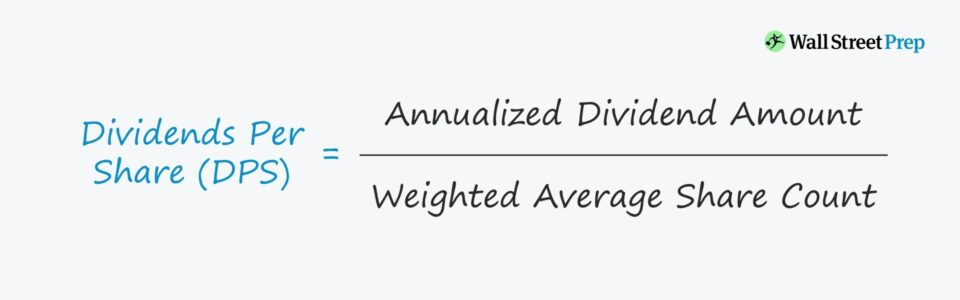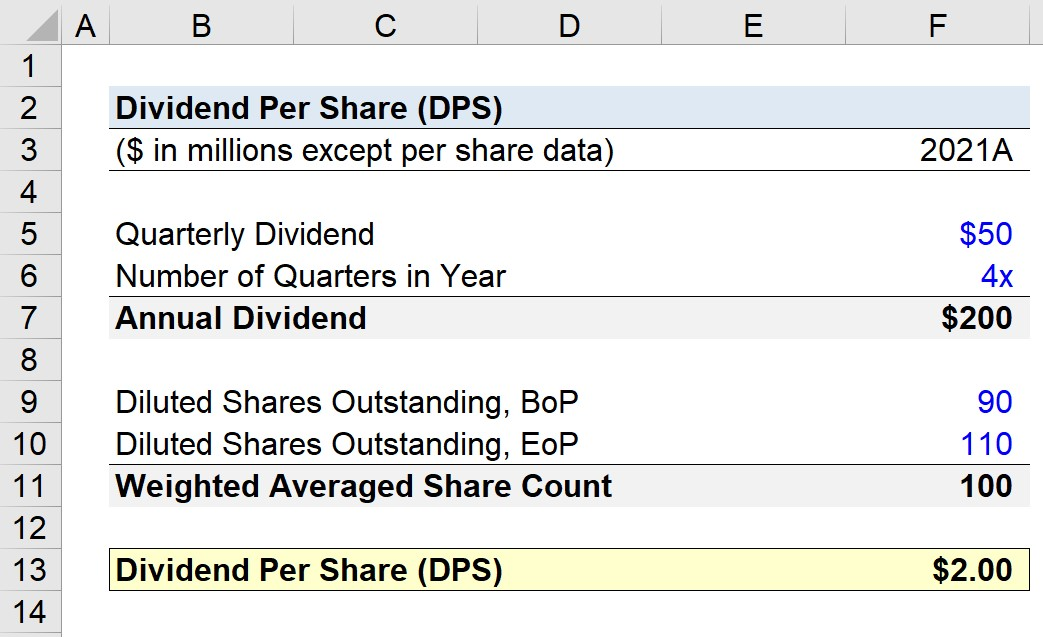# Dividend Per Share (DPS)

Guide to Understanding Dividend Per Share (DPS)## How to Calculate Dividend Per Share (Step-by-Step)

Dividends are defined as the distribution of a company’s after-tax earnings (i.e. net income) to common and preferred shareholders as a form of shareholder compensation.

A common metric used to assess a company’s dividend policy on a per-share basis is the dividends per share (DPS), which standardizes the metric to allow for comparisons in dividend policies among different companies.

Unlike the gross dividend amount, the DPS of a company can also be compared to that of historical periods to observe year-over-year (YoY) trends.

## Dividend Per Share Formula (DPS)

The dividends per share is equal to the dividend issuance amount divided by the total number of shares outstanding.

Dividends Per Share (DPS) = Annualized Dividend Amount ÷ Number of Shares Outstanding

The dividend issuance amount is typically expressed on an annual basis, meaning that a quarterly dividend amount is multiplied by four (i.e. four quarters in one fiscal year) – assuming that the quarterly dividend amount is to remain unchanged.

• Annualized Dividend = Quarterly Dividend Amount x 4

The total number of shares outstanding should include the impact of dilutive securities, as well as be calculated based on the annual weighted average share count between the beginning and end of period shares outstanding.

• Weighted Average of Shares Outstanding = (Beginning and End of Period Shares Outstanding) ÷ 2

## What is a Good Dividend Per Share?

If a company’s dividend per share (DPS) increases, the market reaction is usually positive.

Upon announcing a dividend, especially if a long-term dividend program rather than a one-time issuance, the share price of the underlying issuer tends to rise.

Why? The decision to issue dividends stems from management’s confidence in the company’s future profitability and maintenance of its current market positioning.

By contrast, the cutting of the dividend per share (DPS) sends a negative signal to the market, indicative of uncertainty in the company’s future profits and stability.

However, the context surrounding the issuance of a high dividend per share (DPS) must be considered.

For instance, the management team might have mistakenly announced an unsustainable dividend program, which it refuses to reduce (or end) to avoid sending a negative signal to the market.

In contrast, the decision by a corporation to issue dividends could cause the share price to decline in certain instances. For example, if a company with an inflated share price from its positive growth prospects were to suddenly issue dividends rather than reinvest capital (or participate in a stock buyback), the existing investor base could start to sell-off their positions.

## Dividend Per Share Calculator – Excel Model Template

We’ll now move to a modeling exercise, which you can access by filling out the form below.Submitting ...

## Dividend Per Share Calculation Example (DPS)

Suppose a company issued a quarterly dividend of \$50 million, with no announcements regarding cutting the dividend in the near term.

The annualized dividend amount is calculated to be \$200 million.

• Annual Dividend Amount = \$50 million x 4 = \$200 million

Next, if the company is projected to have 90 million shares at the beginning of the period and 110 million shares outstanding at the end of the period, the weighted average share count is 100 million.

Given those two inputs, if we divide the annualized dividend by the weighted average share count, we calculate \$2.00 as the DPS.

• Dividends Per Share (DPS) = \$200 million ÷ 100 million = \$2.00Step-by-Step Online Course

### Everything You Need To Master Financial Modeling

Enroll in The Premium Package: Learn Financial Statement Modeling, DCF, M&A, LBO and Comps. The same training program used at top investment banks.